# Coax Loss Calculations

Click here to go to a more exact metal loss calculation for coax!

Click here to review the derivation for coax loss due to loss tangent

Click here to go to our main page on transmission line losses

Be sure to visit our "more exact solution" page on the loss of coax due to metal. On this page we will review the simple math for calculating the RF losses of coaxial transmission lines over frequency. There are actually three loss mechanisms that can occur within coax which are each described below:

Calculating coax loss due to metal

RG6 CATV coax cable: an example

Calculating coax loss due to dielectric loss tangent

Calculating loss due to dielectric conductivity (now a separate page)

For reference, the figure below defines two important dimensions of coax, D and d. Note that "D" is the inner diameter of the outer conductor, not its outer diameter!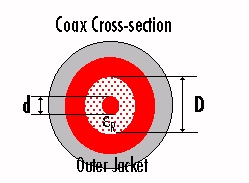## Calculating coax loss due to metal

Update September 2006: we've added a new page that gives a more exact calculation of coax metal loss, which is needed in the case that the skin depth is NOT small compared to to the coax cross section dimensions. In 99.99% of loss calculations, this is probably just an esoteric exercise, but we'll keep it here for you in any case.

Below is the "classic" loss due to metal calculation that you will find in microwave textbooks. Note: this analysis assumes that the skin depth is much smaller than the diameter of the coax center conductor or the thickness of its outer conductor.

Transmission line loss due to metal calculation can be done in a simple three-step process:

1. Calculate RF sheet resistance of conductors.
2. Calculate ohms/length of the geometry.
3. Calculate the loss/length. All of these quantities are functions of frequency.

Step one: calculate RF sheet resistance. This is a function of the metal's permeability and conductivity (in addition to frequency):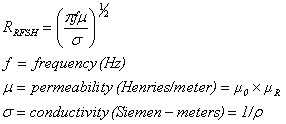Note that you could easily substitute resistivity instead of conductivity into the equation. Click here to look up resistivity of a variety of metals. Don't assume that the inner and outer conductors are made of the same metal, often they are not!

Step two: calculate the resistance per unit length. We recommend you use meters for length to cut down on confusion, but you can use Potrzebies for all we care (pronounced po-SHEB-yas). For coax that has inner conductor diameter "d" and outer conductor inside-diameter "D", you need to integrate the sheet resistance over the "widths" of both cylindrical surfaces:

Resistance/length =(RF sheet resistance)/[(1/πd)+(1/πD)]

Relax, that integration didn't take more than 8th grade math! The "width" of the inner conductor surface is πd and the outside conductor surface is πD. This solution assumes that five skin depths of metal are available on both inner and outer conductor, and each is comprised of a single metal within those five skin depths, which is usually the case. Here's what you get when you plug in RRFSH and simplify: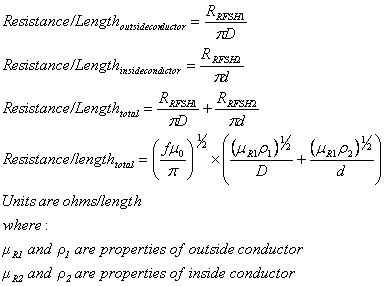Check it out, our equation will let you use different metals for inner and outer conductors, we've never seen such a beautiful sight in any textbook! Or in Agilent's ADS!

The final step is to divide the resistance/length by (2Z0) to arrive at loss/length (units are Nepers/length. To convert to dB, multiply Nepers by 8.686.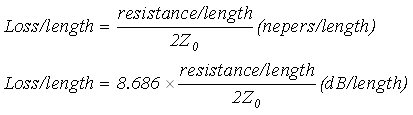The whole ball of wax boils down into the following totally cool closed-form equation: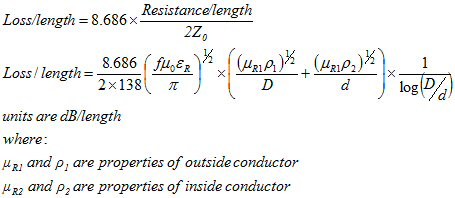Note: Roger pointed out a slight mistake in the above equation in October 2009. Thanks! And sorry for the inconvenience to everyone else!

Now you can calculate the loss/length of any coax, using different metals for inside and outside conductors. Sweet! You can skip the three steps we proposed, and cut to the chase.

### Note: this example examines the only metal loss, not dielectric loss tangent loss...

Let's use RG6 coax cable (which you can buy at Home Depot for wiring up your satellite dish etc.) as an example and see if the math works out close to measured data. Back in 2006 or so, we downloaded a data sheet on this cable to use as a reference for the calculations. You can see it here. We hope CommScope doesn't mind! According to this supplier, the dimensions of RG6 are:

Inside conductor is 18 gauge copper-clad steel (40.4 mils on the AWG scale)

Outside of dielectric is 180 mils diameter

Characteristic impedance is 75 ohms nominal

Outside conductor is aluminum foil.

The dielectric material is "foam polyethylene". Polyethylene by itself has εR=2.25, but in this case it is shot full of air to make it easier to bend, so we don't know off hand what the correct εR is (it must be somewhere between 1 and 2.25, right?

Let's calculate it. We know that the the impedance of RG6 is 75 ohms, and it must obey the coax equation: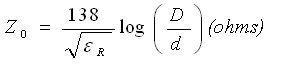The 75 ohm solution is that foam polyethylene εR is 1.43.

Now we calculate the RF sheet resistance of the inner and outer conductors. We did this in a spreadsheet, as a function of frequency. We looked up the conductivities of copper and aluminum here and here. RG6 is supposed to work up to 3 GHz, so we analyzed it up to this frequency.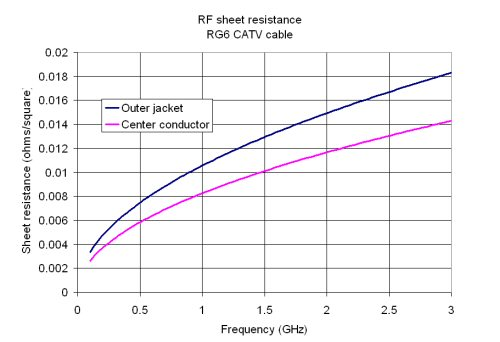Note that the inner conductor has lower sheet resistance. That's because it benefits from the superior conductivity of copper, while the outer jacket is aluminum. We are assuming that the copper-cladding on the steel center conductor is at least 5 skin depths across this frequency band... that assumption might be optimistic at MHz frequencies, but CommScope doesn't provide plating thickness on their data sheet.

Now we calculate the resistance per unit length (ohms per meter). Here the inner conductor contributes the most resistance (even though it has better conductivity), because its surface area is much smaller than the outer conductor. Thanks to Marat for correcting the previous statement!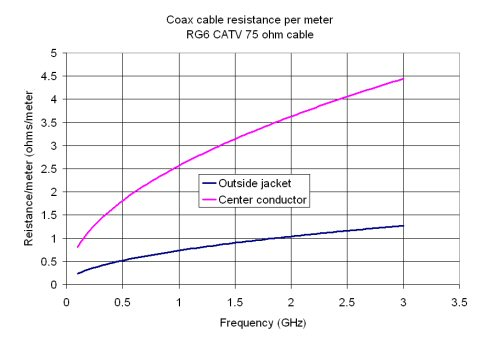Last, we convert to dB/length. We scaled the length units to 100 feet, because most U.S. cable vendors quote RG6 cable loss this way: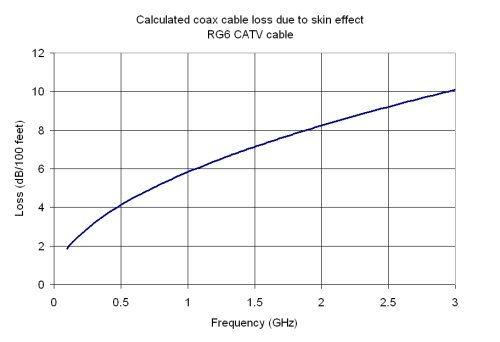So how did the calculation check with real life data? We calculate 5.85 dB per 100 feet at 1 GHz, the supplier says 6.15 dB. That's close enough for government work! Part of the disparity could be that we neglected to look at the effect of dielectric loss tangent loss. But our down-loadable coax spreadsheet will do this for you!

### A possible shortcut...

This info was provided to us by Jorge who works for a large defense contractor. We haven't tried it yet. It relies on obsolete military specification MIL-C-17.

Instead of calculating the conductor and dielectric attenuation using the given formulas on the coax page, you can get the results using:

a = K1 x sqrt(F) + K2 x F (dB/100 feet)

Where K1 is the resistive loss constant
K2 is the dielectric loss constant
F frequency in MHz

Just divide the results by 1200, the number of inches in 100 ft., to get dB/inch. K1 and K2 are available on MIL-C-17 Attenuation and Power Handling tables.

Times Microwave references this calculation on their web site, but they seem to be the only cable supplier that uses it. We couldn't find K1 and K2 for RG6, to compare with the loss calculation we performed.

Good luck!

## Calculating coax loses due to dielectric loss tangent

New for October 2006: the formulas for coax loss due to loss tangent are derived on this separate page.

Loss due to dielectric loss tangent is a pretty easy calculation. The permittivity of a material is actually a complex number, so "epsilon" is made up of two parts: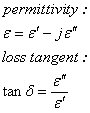Thanks to Paolo for correcting this formula, epsilon double-prime goes in the numerator, not the denominator! Epsilon single-prime is the number we usually deal with, and causes no loss, and in most day-to-day engineering you don't see the prime notation. The imaginary epsilon double-prime is the culprit. Microwave engineers usually deal with the ratio between the two, which is called tangent delta, or tanD (say "tan-dee"), for short. If tanD is zero, there is no loss due to dielectric. If it is non-zero, it creates a loss that is proportional to frequency (inversely proportional to wavelength), shown in the equation below: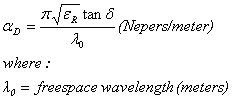Note that in its academic form (above), "natural" units of Nepers/meter result. Also, whatever units you prefer to use for wavelength will be preserved in the loss calculation, so if you enter wavelength in centimeters, the loss will come out in Nepers/centimeter.

In order to arrive at the "more engineeringly" units of decibels/meter, we note that one Neper is 8.68588 dB (exactly 20/ln(10)), and pi=3.14159, then we simplify: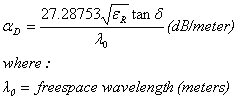In practice, you can round off the constant to 27.3 and not lose sleep. Here's perhaps a handier formula for coax loss due to loss tangent, where you plug in frequency in GHz instead of wavelength: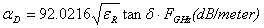By the way, this calculation holds for any TEM transmission line, so it is the same for stripline and rectax! But it won't work for microstrip...

Notice that dielectric loss is proportional to frequency, whereas conductor loss only goes up by the square root of frequency. This goes back to scaling - dielectric loss scales over size and frequency, but conductor loss doesn't. The loss equation also says that you can't reduce dielectric loss by changing the cable geometry, like you can with conductor loss. The only way you can reduce it is to use a dielectric with a very low loss tangent, or low dielectric constant. For example, teflon (a.k.a. PTFE), which is commonly used as a dielectric, has a loss tangent of 0.0004. To illustrate the proportions of conductor and dielectric loss, with a 0.141" diameter semi-rigid cable with copper conductors and Teflon dielectric, the conductor loss is higher than the dielectric loss all the way up to the cutoff frequency, although the two kinds of losses become about equal at the cutoff frequency in this example.

Loss due to dielectric conduction is constant over frequency, and is geometry independent!

Author : Unknown Editor